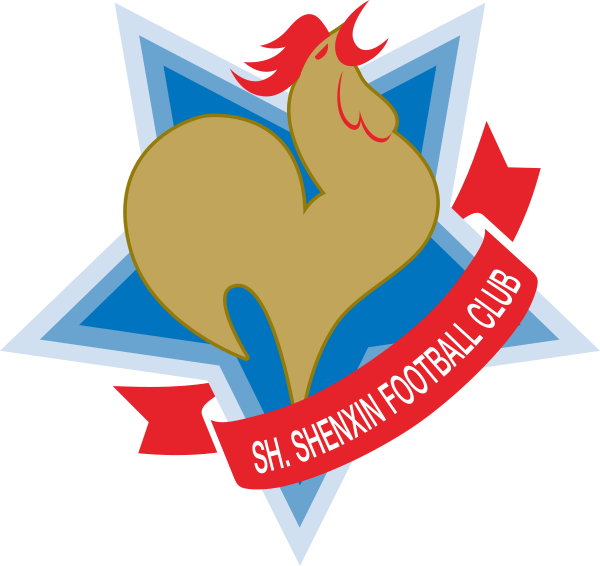# The Color Structure of sp(2N) Gauge Field Theory

For any $T\in \mathfrak{sp}(2N)$ , it is a $(2N)\times (2N)$ matrix constrained by the equation $\Omega T+T^{\mathsf T}\Omega=0,$ where $\Omega=\begin{pmatrix} 0&I_N\\ -I_N&0 \end{pmatrix}.$ Therefore, the dimension of $\mathfrak{sp}(2N)$ is $N(2N+1)$ . If we choose a basis $\{T^a\;:\;1\leq a\leq N(2N+1)\}$ of $\mathfrak{sp}(2N)$ such that $\operatorname{Tr}(T^aT^b)=\delta^{ab},$ then $(T^a)^i_j(T^a)^k_l=\frac{1}{2}\bigl(\delta^i_l\delta^k_j- s(i)s(j)\delta^{\tilde \imath k}\delta_{\tilde \jmath l}\bigr),$ where $\tilde\imath=i+N\in \mathbb Z_{2N}$ and $s(i)=\begin{cases} 1&1\leq i\leq N,\\ -1&N+1\leq i\leq 2N. \end{cases}$ Therefore, $\operatorname{Tr}(AT^a)\operatorname{Tr}(T^aB)= \frac 12\bigl(\operatorname{Tr}(AB)-\operatorname{Tr}(A^{\mathsf T}\Omega^{\mathsf T}B\Omega) \bigr)$ because $(\Omega^{\mathsf T} B\Omega)^i_j=s(i)s(j)B^{\tilde \imath}_{\tilde \jmath}$ . Especially, if $A=T^{a_1}\cdots T^{a_k}$ , then $\operatorname{Tr}(AT^a)\operatorname{Tr}(T^aB)= \frac 12\bigl(\operatorname{Tr}(AB)-(-1)^k\operatorname{Tr}(A_{\text{rev}}B)\bigr),$ where $A_{\text{rev}}=T^{a_k}\cdots T^{a_1}$ . Now take $B=I_N$ , since $\operatorname{Tr}(T^a)=0$ , $\operatorname{Tr}(A)=(-1)^k\operatorname{Tr}(A_{\text{rev}}).$ It’s also true in the case of $\mathfrak{su}(N)$ .

When calculating Feynman diagrams in the usual Yang-Mills theory, we use 3-point vertexs with coefficients $f^{abc}$ and 4-point vertexs to build the gluon amplitude, where $f^{abc}$ is the structure constant of the (semi-simple) gauge group. It can be calculated by the following formula $f^{abc}=\frac{-i}{\sqrt 2}\operatorname{Tr}([T^a,T^b]T^c)$ in our basis. Therefore, for $\mathfrak{sp}(2N)$ gauge field theory, in the calculation of tree level gluon amplitude, the following formula is fundamental: \begin{aligned} f^{a_1a_2\bar a}f^{\bar a a_3 a_4} &=\frac{(-i)^2}{2}\operatorname{Tr}([T^{a_1},T^{a_2}]T^{\bar a})\operatorname{Tr}(T^{\bar a}[T^{a_3},T^{a_4}])\\ &=\frac{(-i)^2}{4}\bigl(\operatorname{Tr}([T^{a_1},T^{a_2}][T^{a_3},T^{a_4}])-(-1)^2 \operatorname{Tr}([T^{a_1},T^{a_2}]_{\text{rev}}[T^{a_3},T^{a_4}])\bigr)\\ &=\frac{(-i)^2}{4}\bigl(\operatorname{Tr}([T^{a_1},T^{a_2}][T^{a_3},T^{a_4}])- \operatorname{Tr}([T^{a_2},T^{a_1}][T^{a_3},T^{a_4}])\bigr)\\ &=\frac{(-i)^2}{2}\operatorname{Tr}([T^{a_1},T^{a_2}][T^{a_3},T^{a_4}]). \end{aligned} Similarly, we can calculate that $f^{a_1a_2\bar a}f^{\bar a a_3 \bar b}f^{\bar b a_4 a_5}= \left( \frac{-i}{\sqrt{2}} \right)^3\operatorname{Tr}\bigl([[T^{a_1},T^{a_2}],T^{a_3}][T^{a_4},T^{a_5}]\bigr)$ and so on. These expressions are both hold for $\mathfrak{su}(N)$ and $\mathfrak{sp}(2N)$ with the different meanings of generators $T^a$ . Therefore, the color structure of $\mathfrak{sp}(2N)$ gauge field theory is the same with $\mathfrak{su}(N)$ in the tree level. However, in the loop level, we may need to calculate $f^{a_1\bar b\bar a}f^{\bar a a_3 \bar b}$ and others which are more complicated, these will be different in the case of $\mathfrak{su}(N)$ .